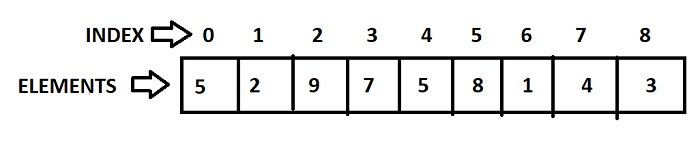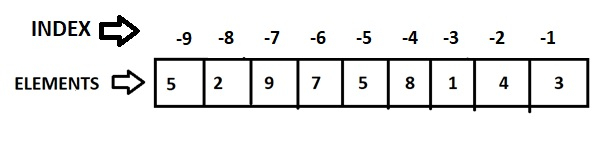# How to index and slice a tuple in Python?

A tuple is an ordered and immutable collection of Python objects separated by commas. Like lists, tuples are sequences. Tuples differ from lists in that they can't be modified, whereas lists can, and they use parentheses instead of square brackets.

tup=('tutorials', 'point', 2022,True)
print(tup)


If you execute the above snippet, produces the following output −

('tutorials', 'point', 2022, True)


In this article, we will discuss how to index and slice tuples in python.

## Indexing Tuples

In Python, every tuple with elements has a position or index. Each element of the tuple can be accessed or manipulated by using the index number.

They are two types of indexing −

• Positive Indexing
• Negative Indexing

### Positive Indexing

In positive the first element of the tuple is at an index of 0 and the following elements are at +1 and as follows.

In the below figure, we can see how an element in the tuple is associated with its index or position.### Example 1

The following is an example code to show the positive indexing of tuples.

tuple= (5,2,9,7,5,8,1,4,3)
print(tuple(3))
print(tuple(7))


### Output

The above code produces the following results

7
4


## Negative Indexing

In negative indexing, the indexing of elements starts from the end of the tuple. That is the last element of the tuple is said to be at a position at -1 and the previous element at -2 and goes on till the first element.

In the below figure, we can see how an element is associated with its index or position of a tuple.### Example

The following is an example code to show the negative indexing in tuples.

tuple= (5,2,9,7,5,8,1,4,3)
print(tuple(-2))
print(tuple(-8))


### Output

The above code produces the following results

4
2


## Slicing tuples

Tuple slicing is a frequent practice in Python, and it is the most prevalent technique used by programmers to solve efficient problems. Consider a Python tuple. You must slice a tuple in order to access a range of elements in it. One method is to utilize the colon as a simple slicing operator (:).

The slice operator allows you to specify where to begin slicing, where to stop slicing, and what step to take. Tuple slicing creates a new tuple from an old one.

### Syntax

tuple[Start : Stop : Stride]


The above expression returns the portion of the tuple from index Start to index Stop, at a step size Stride.

### Example 1

In the following example we have used the slice operation to slice a tuple. We also use negative indexing method to slice a tuple.

tuple= ('a','b','c','d','e','f','g','h','i','j')
print(tuple[0:6])
print(tuple[1:9:2])
print(tuple[-1:-5:-2])


### Output

The above code produces the following results

('a', 'b', 'c', 'd', 'e', 'f')
('b', 'd', 'f', 'h')
('j', 'h')


### Example 2

Following is another example for this −

my_tuple = ('t', 'u', 'r', 'i', 'a', 'l', 's', 'p','o', 'i', 'n', 't')
print(my_tuple[1:]) #Print elements from index 1 to end
print(my_tuple[:2]) #Print elements from start to index 2
print(my_tuple[5:12]) #Print elements from index 1 to index 3
print(my_tuple[::5]) #Print elements from start to end using step size


### Output

('u', 'r', 'i', 'a', 'l', 's', 'p', 'o', 'i', 'n', 't')
('t', 'u')
('l', 's', 'p', 'o', 'i', 'n', 't')
('t', 'l', 'n')


Updated on: 29-Aug-2023

18K+ Views